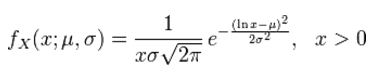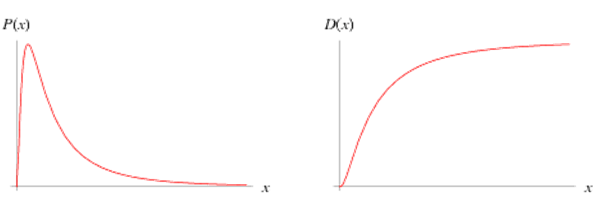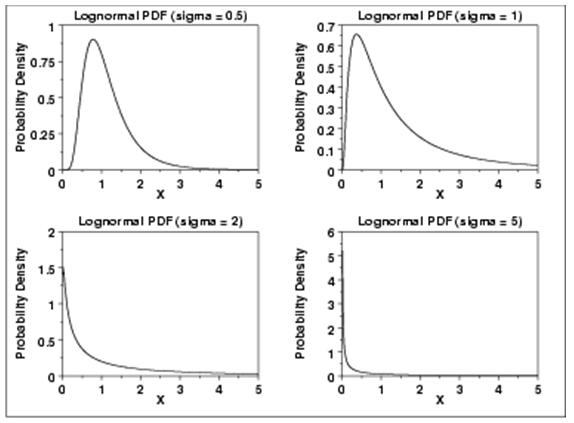# Properties of Log-Normal Distribution

Definition

If the logarithm to the power of the variable x is normally distributed then the variable itself is said to be lognormally distributed. In other words if ln(x) is normally distributed then the variable x is supposed to have a log-normal distribution.

The probability density function for this variable is as follows:In this equation µ and σ are the mean and the standard deviation of the variable’s natural logarithm.

A typical log-normal function looks as depicted in the graph below:The plot of the log-normal distribution for various values of the standard deviation is as below:Occurrence

In financial markets the returns on asset prices are assumed to be normally distributed. If the return is denoted by the following equation:

r = (P1 – P0) / P0

Where P0 and P1 are the prices at time 0 and 1 respectively, then in theory it is possible that P1 might turn out to be negative as r could end up below -1. In order to account for such situations it would be safe to use the log-normal distribution.

If a random variable X is defined as P1/P0 and the logartihm of this variable ln(X) is normally distributed, since X can never be negative it means that P1 can never be negative.

However in the real world it is rare that stock prices are negative as businesses do not have situations where they have large liabilities or outgoing cashflows. Also in practice when the changes in price are small and the time period is not that large the possibility of having a negative price is very small.

Applications

This pattern finds wide application in finance and one of the most famous applications is in the Black-Scholes option pricing model commonly used to value options. This model assumes that the returns of commonly used financial asset values like stock prices or foreign exchange rates or price indices or stock market indices are log-normally distributed. Which means the log of the returns should be normally distributed.

However there have been several situations where this assumption fails as in case of very sudden changes in market factors like in the case of stock market crashes or situations involving economic collapses like the Asian Financial Crisis which saw liquidity drying up in the market rapidly causing dramatic changes in the shape of the yield curve with short term rates suddenly sky rocketing. In this case the distributions develop very fat tails and models other than Black-Scholes like log-levy distributions are used to factor in this effect.